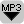## What is Determinism?Determinism refers to the idea that the future is not up to chance, the future can be predicted. Determinists claim that if you had sufficient knowledge of the current situation, then you could in theory predict the behavior of a system into the future. Certain systems are indeed deterministic, many others are not.

In this edition we will have a look at the concept of determinism. In a deterministic system the initial conditions of the system determine the future behavior of the system. The behavior of both a falling apple and a rolling die depend on the initial conditions. Even the same laws of physics apply, namely classical mechanics. Both falling apple and the rolling dice are deterministic systems. But I am able to calculate the landing position of a falling apple, but I am not able to calculate the result of a die roll. Why? How are these two examples different? Why does the die seem to behave according to chance, but not a falling apple? A rolling die is chaotic and it is unpredictable, even though it is deterministic. Even if we know all the physical laws and the starting conditions of a system, it is still not possible to predict the future behavior of chaotic systems.

# Transcript:

In this edition of TOK-Talk we will explore if sciencific laws can predict the future behavior of some systems. What is determinism? Listen to find out!

Two, five, one….. Maybe you can hear it, I am rolling a die. I can not predict the next number, it seems to be – pure chance. But is it chance? The behavior of the die depends on physical laws, so it should be possible to calculate or predict the result with the help of a computer. Probably you say that the result is different because I am not throwing the die in the same way. And you are right. The roll of the die depends on the initial conditions. It depends on the way that it is on my palm, and on the way that I throw the die. We say that the die behaves in a deterministic way. The starting conditions and the rules of physics determine the outcome of the die roll. The die follows a strict cause and effect relationship.

During the 19th century determinism was commonly accepted among the scientists. If we have a full information about the current state of the world, then we can predict the future behavior of the world. The universe, according to this view, works like a clockwork, and even human behavior is deterministic. I once even read that some scientists were frustrated about this, because they thought that there was essentially nothing new to discover anymore. If we know the position and movement of every atom of this world, then it is possible, using Newton’s laws of motion, to predict the future development of the world. We can even predict human behavior this way, we remember that humans are also made of atoms.

Well, this view has changed dramatically during the last century. I want to quote Sir Karl Popper here:

Determinism means that if you have sufficient knowledge of chemistry and physics you can predict what Mozart will write tomorrow. Now this is a ridiculous hypothesis. Sir Karl Popper (1992)

I want to give you two examples why it is not possible to predict the future behavior of some systems. The first example is from chaos theory and the second one is from nuclear physics, from quantum mechanics.

Drawing of the lottery numbers in TV: plastic balls arranged according to number, the plastic balls fall into a container, they are mixed and then the numbers are drawn. And if you are lucky you win. Now why is it that always different numbers are drawn? Should it not be possible, using the equations of physics, and with the help of a computer, to calculate the result? I know the starting positions of the plastic balls, I know their mass, I know how the machine works and how it mixes the balls, and so on. So why is it, that we always get different results? Why can we not compute the ourcome? This is because very, very small deviations in the starting conditions can have large effect at the end. It is impossible to arrange the balls exactly the same way and it is impossible to mix them exactly the same way. The lottery machine is deterministic, but it is also unpredictable – it is a chaotic system. There are of course many other examples of chaotic systems in our daily lives: the weather, the rolling die from the beginning, etc. We can not predict their behavior, even though we know the physical laws involved.

The second example that illustrates why the world is not predictable is quantum mechanics. At an atomic level cause and effect relationships break down. It is, for example, impossible to say when a particular radioactive atom will fall apart. There is no external factor that causes it to fall apart. At an atomic scale the world is not deterministic anymore and we can only use probabilities to describe its behavior.

The world changed from having the determinism of a clock to having the contingency of a pinball machine. Heinz R. Pagels

# Questions for Discussion:

• If human behavior is deterministic (and dependent on biochemical events of the nervous system), then do humans have a free will? How do we know if we have a free will?
• If a theory is not capable of making a specific prediction, due to the chaotic behavior of a system (such as the weather), then can this theory be considered scientific?
• Probabilities play a high importance in modern physics, especially in quantum mechanics (but also chaos theory). Are theories that rely on statistical explanations falsifyable? Or to what extent are they falsifyable? After all, statistical, probabilistic outcomes are not simple yes/no answers.